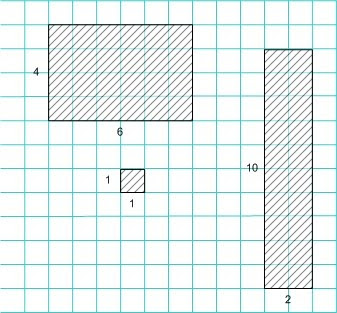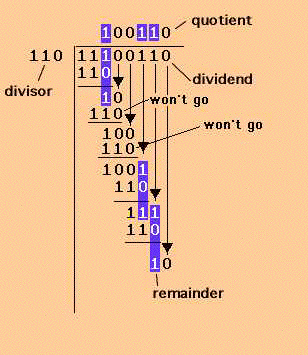## Monday, March 1, 2010

### Binary ArithmeticBoolean variables 0 and 1 form the basis of many complex IC on any of our electronic devices. This post starts with their basics leading to understanding of some basic digital circuits.

Rules of binary addition:

0 + 0 =0
0 + 1 = 1
1+ 0 = 1
1 + 1 = 0 (with a carry of 1)
1+ 1 + 1 = 10 + 1 = 11
Rules of binary subtraction:

0- 0 =0
1 - 0 = 11 -1 = 0

0 -1 = 1 (with a borrow of 1)Rules of binary multiplication:

Repeated addition of one number by times equal to other number

Rules of binary division:x / y implies count the number of times y can be subtracted from x with positive or zero remainder.

Multiplication of two given floating point numbers is carried out by multiplying their mantissas and adding the exponent values on a common base.

Division of two given floating point numbers is carried out by dividing the dividend mantissa by the divisor mantissa and subtracting their common base exponent values. Normalize the result if required.

Combination of different binary variables gives rise to LOGIC Gates. Each of the basic logic gates is a piece of hardware or an electronic circuit that can be used to implement the most basic logical expressions. 3 basic self explanatory logic gates are AND OR NOT

For Ex-OR gate, output equals 0 for equal inputs and 1 for unequal inputs.

The output of NAND gate is 0 when all the inputs are 1.

The output of NOR gate equals 1 when all its inputs are 0.

NAND and NOR gates have a special property that these gates can individually be used to construct logic circuit for any boolean expression making them universal gates.

Quiz:
1. How many binary digits can be added using a half adder circuit?

2. A logic gate with 4 inputs can give how many possible input combinations?

3. NOT gate can have how many input variables?

4. What is the resultant gate f ormed by shorting all the inputs of a NOR gate?

5. Is 10110001 necessarily a binary number?

Sandy said...

Wonderful Tee comment! Really funny.

I could not understand the last question, though? Am sure I am missing something very basic here! Would you mind letting me know of it.

Pilot-Pooja said...

Thats the only trick Sandy!
I meant in how many languages this notation is possible?Anonymous said...

Nice post and this post helped me alot in my college assignement. Gratefulness you seeking your information.

Mindbox